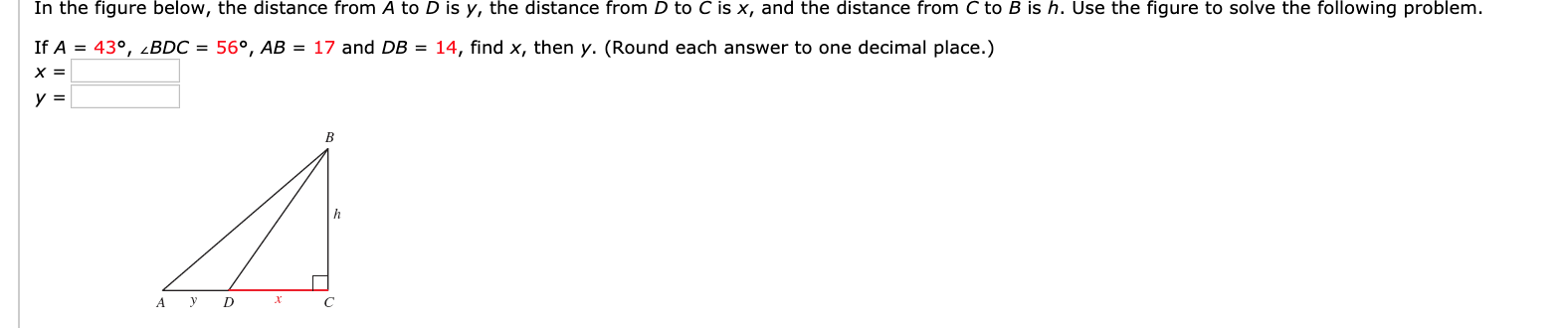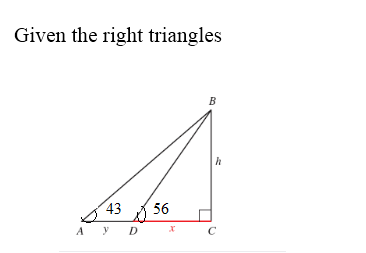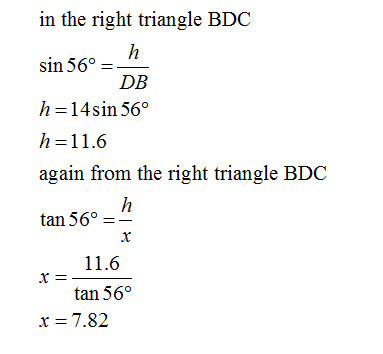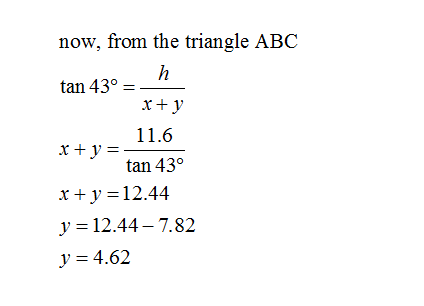# In the figure below, the distance from A to D is y, the distance from D to C is x, and the distance from C to B is h. Use the figure to solve the following problem.If A = 43°, 2BDC = 56°, AB = 17 and DB = 14, find x, then y. (Round each answer to one decimal place.)х 3BD

Question
2 views

Solving Right Triangleshelp_outlineImage TranscriptioncloseIn the figure below, the distance from A to D is y, the distance from D to C is x, and the distance from C to B is h. Use the figure to solve the following problem. If A = 43°, 2BDC = 56°, AB = 17 and DB = 14, find x, then y. (Round each answer to one decimal place.) х 3 B D fullscreen
check_circle

Step 1Step 2Step 3...

### Want to see the full answer?

See Solution

#### Want to see this answer and more?

Solutions are written by subject experts who are available 24/7. Questions are typically answered within 1 hour.*

See Solution
*Response times may vary by subject and question.
Tagged in